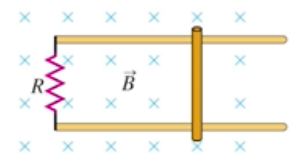Need Help?

Subscribe to Physics 2

###### \${selected_topic_name}
• Notes
• Comments & Questions

$\begin{array}{l}{\text { Consider the circuit shown in Fig. } E 29.29 \text { , but with the }} \\ {\text { bar moving to the right with speed } v . \text { As in Exercise } 29.29 \text { , the bar }} \\ {\text { has length } 0.360 \mathrm{m}, R=45.0 \Omega, \text { and } B=0.650 \mathrm{T} \text { . (a) Is the }} \\ {\text { induced current in the circuit clockwise or counterclockwise? }} \\ {\text { (b) At an instant when the } 45.0-\Omega \text { resistor is dissipating electrical }} \\ {\text { energy at a rate of } 0.840 \mathrm{J} / \mathrm{s}, \text { what is the speed of the bar? }}\end{array}$

$P_{R}=I^{2} R$

$\varepsilon=B V L$

$I=\frac{\varepsilon}{R}$

(a) $\text { Clockwise. }$

(b) $\text { I?? }$$\longrightarrow P_{R} = I^{2} R \rightarrow I=\sqrt{\frac{P_{r}}{R}}$

$=\sqrt{\frac{0.84 w}{45}}=0.1366 A$

$V=\frac{I R}{B L}=\frac{0.1366 * 45}{0.65 * 0.36}=$$26.3 \mathrm{m/S} \approx 60 \mathrm{mph}$

$\begin{array}{l}{\text { A } 0.250 \text { -m-long }} \\ {\text { moves on parallel rails that are }} \\ {\text { connected through a } 6.00-\Omega} \\ {\text { resistor, as shown in Fig. } \mathrm{E} 29.31 \text { , }} \\ {\text { so the apparatus makes a complete }} \\ {\text { circuit. You can ignore the resist- }} \\ {\text { ance of the bar and rails. The cir- }} \\ {\text { cuit is in a uniform magnetic field }}\end{array}$

$\begin{array}{l}{B=1.20 \mathrm{T} \text { that is directed into the plane of the figure. At an }} \\ {\text { instant when the induced current in the circuit is counterclockwise }} \\ {\text { and equal to } 1.75 \mathrm{A}, \text { what is the velocity of the bar (magnitude and }} \\ {\text { direction)? }}\end{array}$$\varepsilon=B VL$

$I=\frac{\varepsilon}{R}$

$I=\frac{\varepsilon}{R}=\frac{B V L}{R} \rightarrow I=\frac{B V L}{R}$

$V=\frac{I{R}}{B L}=\frac{1.75*6}{1.2 * 0.25}=35 \mathrm{m/s}$

No comments yet